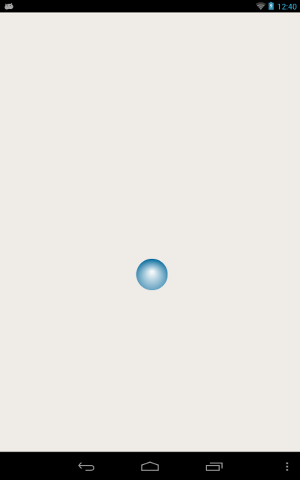# Qt Sensors - Accel Bubble

The AccelBubble example demonstrates the Accelerometer QML type.#### Overview

Writing a QML application that uses the Accelerometer QML sensors type requires the following steps:

Import the Sensors Declarative module.

```
import QtSensors 5.0
```

```
Accelerometer {
id: accel
dataRate: 100
```

Use the 'active' property to start the sensor

```
active:true
```

Move the bubble according to a factor of the accelerator sensor

```

if (isNaN(newX) || isNaN(newY))
return;

if (newX < 0)
newX = 0

if (newX > mainWindow.width - bubble.width)
newX = mainWindow.width - bubble.width

if (newY < 18)
newY = 18

if (newY > mainWindow.height - bubble.height)
newY = mainWindow.height - bubble.height

bubble.x = newX
bubble.y = newY
}
```

Example project @ code.qt.io